## Overview🔗

Flow physics:

• Internal flow
• Moderate Reynolds number
• Spanwise direction: Statistically homogeneous
• Streamwise and channel-height directions: Statistically developing
• Newtonian, single-phase, incompressible, non-reacting

Solver:

Tutorial case:

## Physics and Numerics🔗

Physical domain:

• The case is a statistically-developing internal flow through parallel smooth walls which are two characteristic-length apart.
• $$x$$: Longitudinal direction (Mean-flow direction)
• $$y$$: Vertical direction (Wall-normal direction)
• $$z$$: Spanwise direction (Statistically homogeneous direction)
• $$O$$: Origin at the left-bottom corner of the numerical domain

Physical modelling:

• Reynolds number based on friction velocity: $$\text{Re}_{u_\tau} = \vert \mathbf{U}_\tau \vert \delta / \nu_\text{fluid} = 395$$ [-]
• (Estimated) Friction velocity: $$\mathbf{U}_\tau = (1.0, 0.0, 0.0)$$, and $$\vert \mathbf{U}_\tau \vert = u_\tau = 1.0$$ [m⋅s-1]
• Characteristic length (Channel half-height): $$\delta = 1.0$$ [m]
• Kinematic viscosity of fluid: $$\nu_{\text{fluid}} \approx 0.002532$$ [m2⋅s-1]
• Bulk velocity of flow: $$\mathbf{U}_b = (17.55, 0.00, 0.00)$$ [m⋅s-1]
• Turbulence model: Large eddy simulation with the Smagorinsky sub-filter scale model utilising the van Driest wall-damping function. The sub-filter scale model constants:
• $$C_k \approx 0.02655$$
• $$C_e = 1.048$$
• $$C_s \approx 0.065$$
• $$C_s = (C_k \{C_k/C_e\}^{0.5} )^{0.5}$$

Numerical domain modelling:

• Shape: Rectangular prism
• Dimensions: $$(x, y, z) = (20.0\pi, 2.0, \pi)$$ [m]
• Sketch: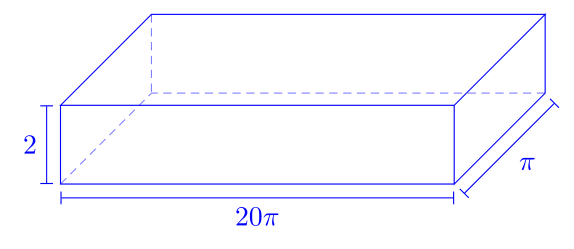Spatial domain discretisation:

• Mesh type: Rectangular cuboid mesh
• Mesher: blockMesh
• Number of nodes, $$N$$: $$(N_x, N_y, N_z) = (500, 46, 82)$$ [nodes]
• Spatial resolution $$(\Delta)$$ distribution:
• Uniform in $$(x, z)$$-directions
• Stretched in $$(y)$$-direction; clustered nearby walls
• Uniform mesh particulars:
• $$\Delta_x^+ = (\Delta_x u_\tau )/\nu_{\text{fluid}} \approx 49.6$$ [-]
• $$\Delta_z^+ \approx 15.1$$ [-]
• Wall-normal mesh particulars:
• Simple grading expansion ratio: 25.0 [-] (From top to bottom wall, the ratio is 0.04)
• First wall-normal node height: $$\Delta_y^+ \approx 1.1$$
• Mesh details: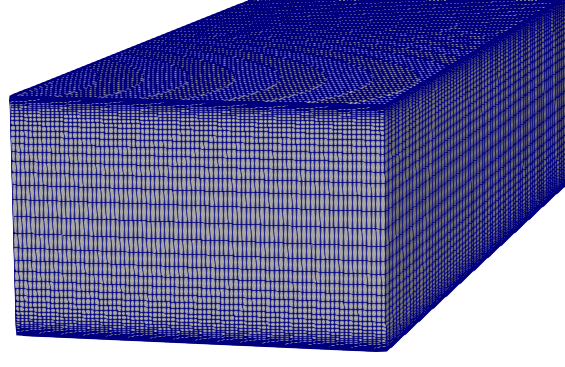Temporal domain discretisation:

• Time-step size: $$\Delta_t = 0.004$$ [s]
• Estimated Courant-Friedrichs-Lewy (CFL) number based on $$\{ \overline{u_x} \}_{y^+ = 392} = 20.133$$[m⋅s-1]: CFL $$\approx 0.64$$

Equation discretisation:

Spatial derivatives and variables:

• Divergence: Gauss linear
• Laplacian: Gauss linear orthogonal

Temporal derivatives and variables:

Numerical boundary conditions:

• Velocity, $$\mathbf{U}$$
Patch Condition Value [m⋅s-1]
Inlet turbulentDFSEMInlet (17.55, 0.00, 0.00)
Outlet inletOutlet (0.0, 0.0, 0.0)
Sides ($$z$$-dir) cyclic -
Walls ($$y$$-dir) fixedValue (0.0, 0.0, 0.0)
• Pressure, p
Patch Condition Value [m2⋅s-2]
Outlet fixedValue 0.0
Sides ($$z$$-dir) cyclic -
Walls ($$y$$-dir) zeroGradient -
• Turbulent kinematic viscosity, nut (i.e. $$\nu_t$$)
Patch Condition Value [m2⋅s-1]
Inlet calculated -
Outlet calculated -
Sides ($$z$$-dir) cyclic -
Walls ($$y$$-dir) zeroGradient -

Solution algorithms and solvers:

• Pressure-velocity: PISO
• Parallel decomposition of spatial domain and fields: scotch
• The bandwidth of the coefficient matrix is minimised by renumberMesh
• Linear solvers:
Field Linear Solver Smoother Relative Tolerance
U smooth GaussSeidel 0.0
p GAMG GaussSeidel 0.0
nuTilda smooth GaussSeidel 0.0

Initialisation and sampling:

• Computation time for a single domain pass-through based on $${ \overline{U_x} }_{y^+ = 392} = 20.133$$) [m2⋅s-1] $$\approx 3.121$$ [s]
• Initialisation pass-throughs = $$\approx 2.7$$ 
• Sampling pass-throughs = $$\approx 24.5$$ 

## Results🔗

List of metrics:

• Prescribed vs. reproduced Reynolds stress tensor components at inlet patch
• $$\overline{u^\prime u^\prime}$$ downstream development vs. $$x/ \delta$$
• $$\overline{v^\prime v^\prime}$$ downstream development vs. $$x/ \delta$$
• $$\overline{u^\prime v^\prime}$$ downstream development vs. $$x/ \delta$$
• Surface skin friction coefficient $$\mathrm{C}_f$$ vs. $$x/ \delta$$
• Streamwise mean flow speed and Reynolds stress tensor components at uniform-interval downstream profiles
• Streamwise vorticity $$\omega_x$$ at $$x/ \delta = 0.1$$
• Streamwise vorticity $$\omega_x$$ at $$x/ \delta = 1.0$$
• Metrics are time and spanwise averaged
• $$< \cdot >$$ is the time-averaging operator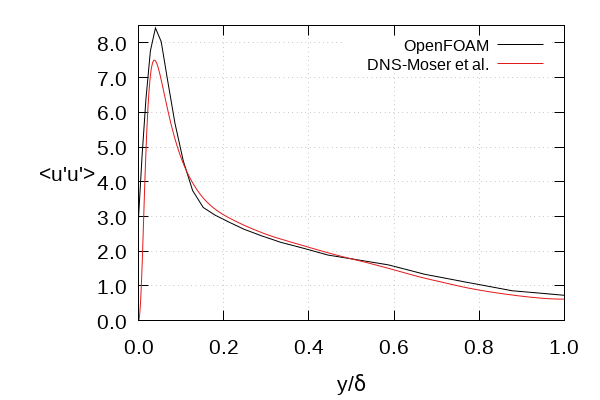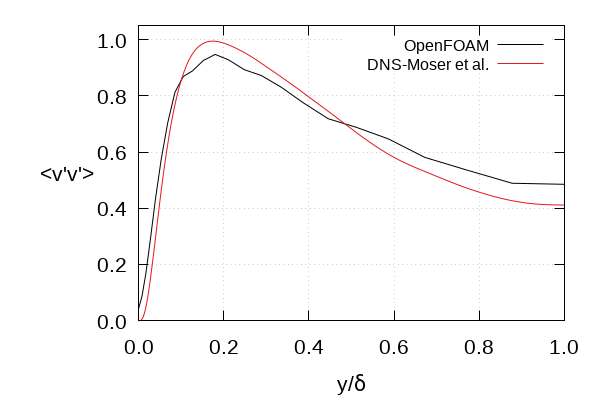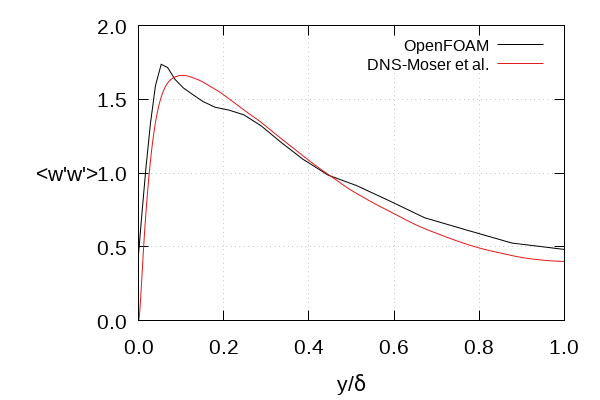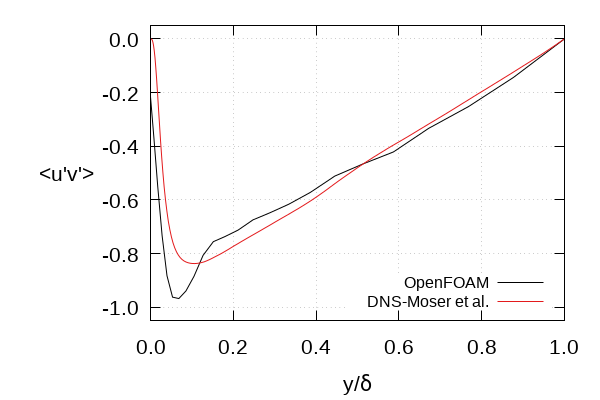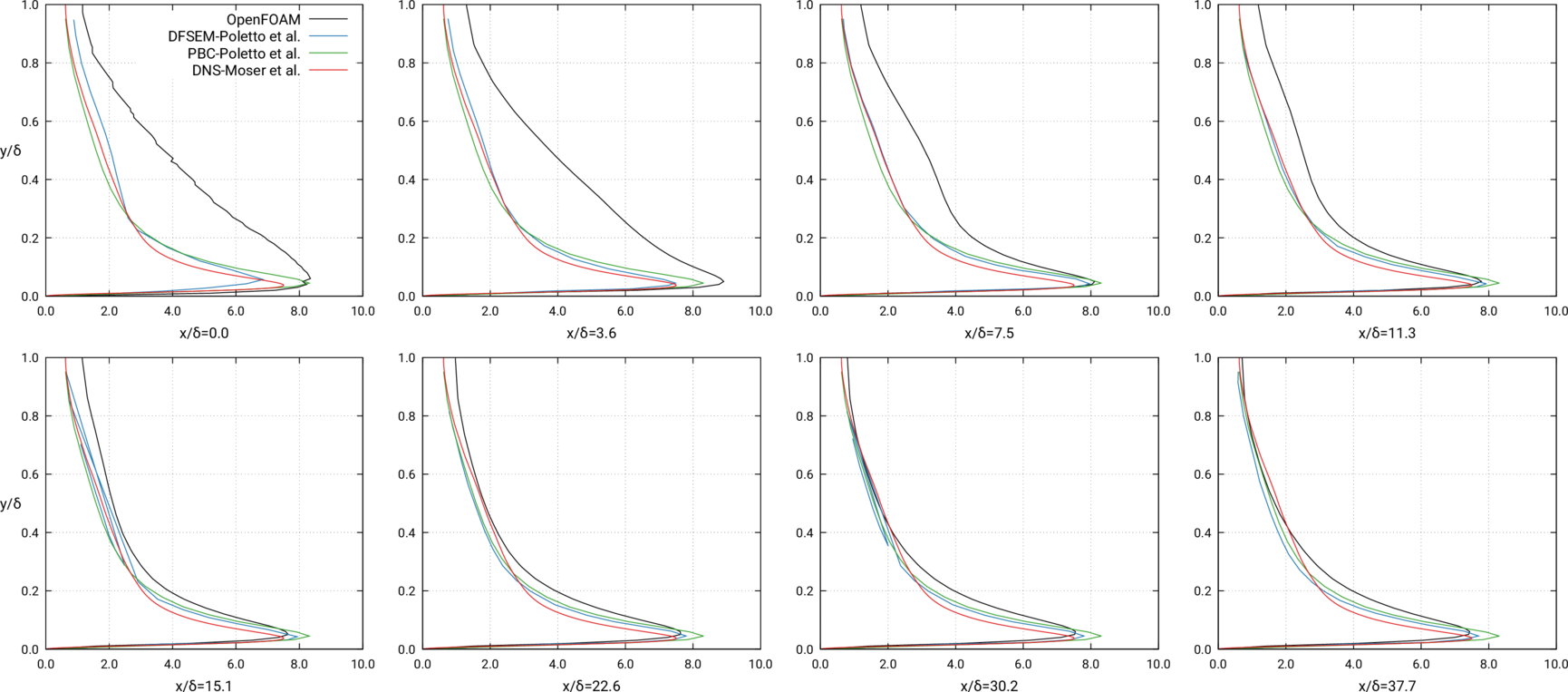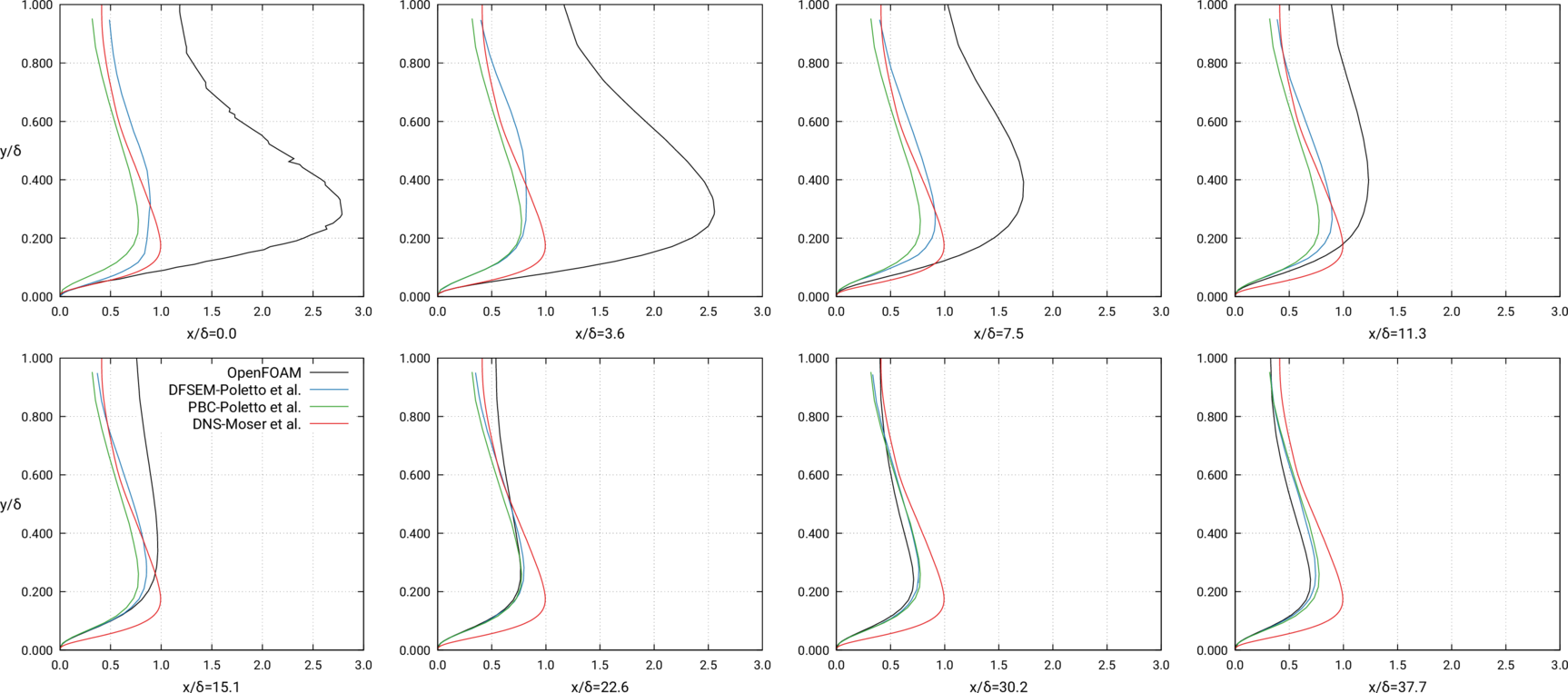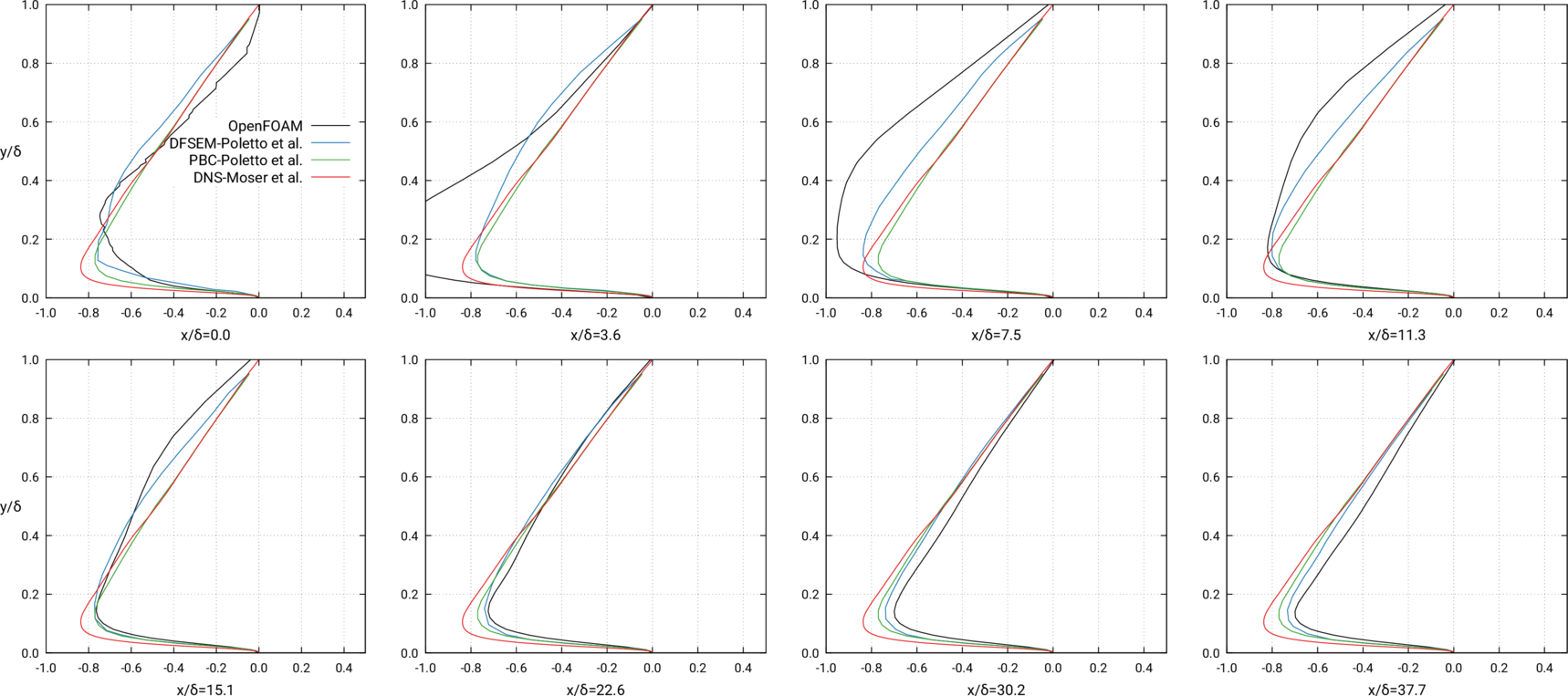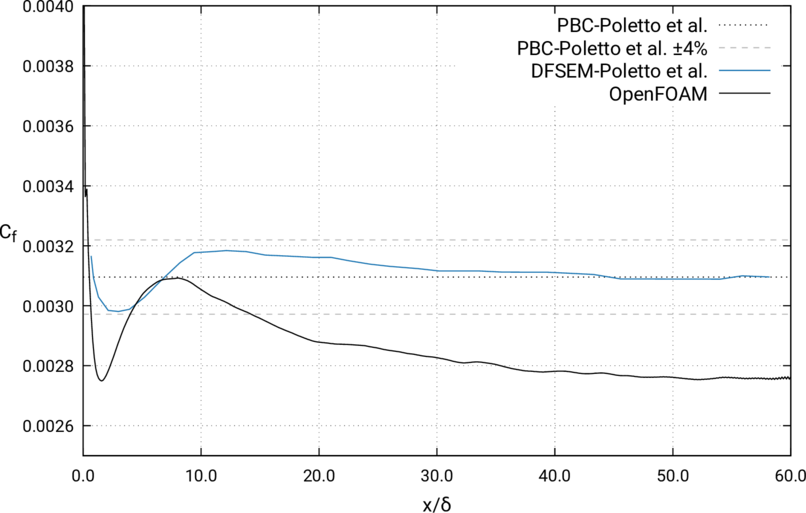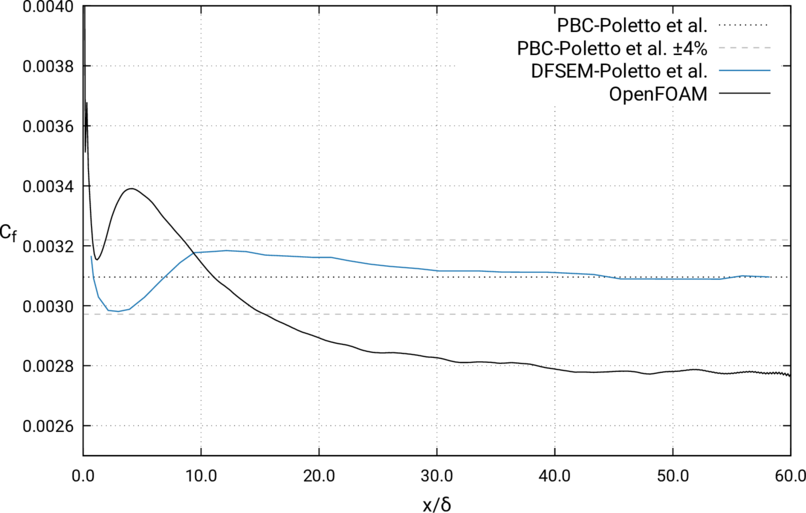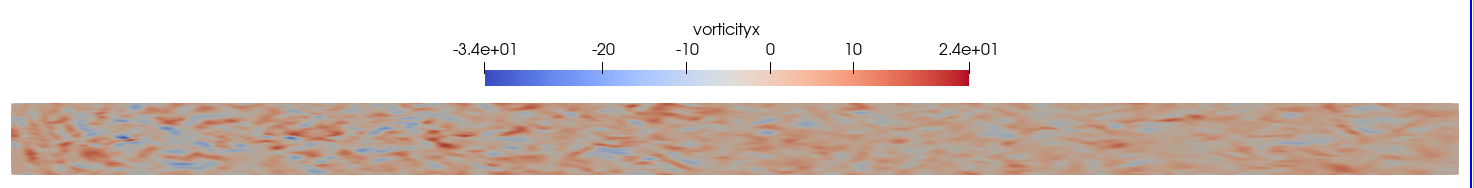## Resources🔗

Note: Links will take you to the University of Texas at Austin website

### Datasets for verifications (plain text)🔗

Reynolds stress tensor profiles:

Mean velocity profiles:

Two-point velocity correlations (i.e. Auto- and cross-correlation functions):